John Zhu

Parametric Curves

Slide Duration:

Section 1: Prerequisites
Parametric Curves

10m 10s

Intro
0:00
Parametric Equations
0:23
Familiar Functions
0:32
Parametric Equation/ Function
0:56
Example 1: Graph Parametric Equation
1:48
Example 2
4:30
Example 3
6:01
Example 4
7:12
Example 5
8:10
Polar Coordinates

14m 54s

Intro
0:00
Polar Coordinates
0:09
Definition
0:11
Example
0:23
Converting Polar Coordinates
1:49
Example: Convert Polar Equation to Cartesian Coordinates
3:06
Example: Convert Polar Equation to Cartesian Coordinates
5:21
Example: Find the Polar Representation
6:51
Example: Find the Polar Representation
8:39
Example: Sketch the Graph
10:02
Vector Functions

12m 5s

Intro
0:00
Vector Functions
0:11
2 Parts
0:18
Example 1
1:44
Example 2
3:52
Example 3
4:59
Example 4
6:03
Example 5
8:00
Example 6
9:23
Example 7
10:16
Section 2: Differentiation
Parametric Differentiation

13m 15s

Intro
0:00
Parametric Differentiation
0:15
Example 1
1:16
Example 2
1:54
Example 3
3:15
Example 4
4:59
Parametric Differentiation: Position, Speed & Acceleration
7:45
Example 5
8:32
Example 6
10:37
Polar Differentiation

10m 14s

Intro
0:00
Polar Differentiation
0:11
Goal
0:13
Method
0:24
Example 1
0:52
Example 2
4:27
Example 3
7:03
L'Hopital's Rule

6m 43s

Intro
0:00
L'Hopitals Rule
0:09
Conditions
0:14
Limit
0:36
Example 1
1:21
Example 2
2:38
Example 3
4:09
Example 4
5:01
Section 3: Integration
Integration By Parts

19m 4s

Intro
0:00
Integration by Parts
0:12
Formula
0:31
How It's Derived From Product Rule
1:09
Integration by Parts: Rules to Follow
3:24
Recap of the Rules
4:13
Example 1
4:29
Example 2
7:04
Example 3
8:40
Example 4
13:48
Example 5
15:36
Integration By Partial Fractions

22m 4s

Intro
0:00
Integration by Partial Fractions: Goal
0:08
Example 1
2:10
Example 2
7:10
Example 3
10:06
Example 4
14:23
Example 5
16:26
Improper Integrals

19m 1s

Intro
0:00
Improper Integrals
0:06
3 Steps
1:11
Example 1
1:46
Example 2
3:20
Example 3
6:05
Example 4
9:02
Example 5
11:21
Example 6
15:54
Section 4: Applications of Integration
Logistic Growth

19m 16s

Intro
0:00
Logistic Growth Function
0:07
Defining Variables
0:40
Equation Parts
1:51
Logistic Growth
2:04
Example 1
2:59
Example 2
7:13
Example 3
11:29
Example 4
15:21
Arc Length for Parametric & Polar Curves

17m 40s

Intro
0:00
Arc Length
0:13
Arc Length of a Normal Function
0:24
Example
1:27
Example 2: Arc Length for Parametric Curves
3:31
Example 3: Arc Length for Parametric Curves
4:23
Example 4: Arc Length for Parametric Curves
8:05
Example 5: Arc Length for Parametric Curves
12:22
Example 6: Arc Length for Polar Curves
15:36
Example 7: Arc Length for Polar Curves
16:03
Area for Parametric & Polar Curves

4m 33s

Intro
0:00
Area for Parametric Curves: Parametric Function
0:10
Example 1: Area for Parametric Curves
0:35
Area for Parametric Curves: Polar Function
2:50
Example 2: Area for Polar Curves
3:18
Section 5: Sequences, Series, & Approximations
Definition & Convergence

7m 10s

Intro
0:00
Sequences: Definition
0:09
Definition
0:31
Example 1
1:07
Sequences: Convergence
2:02
Example 1
2:52
Example 2
3:36
Example 3
4:47
Example 4
6:16
Geometric Series

10m 59s

Intro
0:00
Geometric Series
0:07
Definition
0:24
Expanded Form
0:39
Convergence Rules
1:00
Example 1: Convergence
1:22
Example 2: Convergence
2:36
Example 3: Convergence
3:45
Sum of Series
5:04
Sum of First n Terms
5:14
Sum of Series
5:24
Example 1: Sum of Series
5:39
Example 2: Sum of Series
7:15
Example 3: Sum of Series
8:24
Example 4: Sum of Series
9:36
Harmonic & P Series

3m 58s

Intro
0:00
Harmonic Series
0:08
P-Series
1:17
Example 1: P-Series Test
2:22
Example 2: P-Series Test
3:06
Integral Test

11m 31s

Intro
0:00
Integral Test
0:09
Example 1
0:54
Example 2
4:00
Example 3
7:59
Example 4
9:47
Comparison Test

7m 47s

Intro
0:00
Comparison Test
0:10
Example 1
1:07
Example 2
2:33
Example 3
4:20
Example 4
6:29
Limit Comparison Test

9m 40s

Intro
0:00
Limit Comparison Test
0:09
Conditions
0:31
Example 1
1:01
Example 2
2:53
Example 3
4:15
Example 4
6:19
Ratio Test

11m 20s

Intro
0:00
Ratio Test
0:09
Example 1
0:57
Example 2
2:55
Example 3
6:27
Example 4
8:36
Alternating Series

9m 11s

Intro
0:00
Alternating Series
0:08
Convergence Test
0:59
Example 1
1:27
Example 2
2:42
Example 3
4:57
Example 4
6:37
Absolute Convergence

7m 13s

Intro
0:00
Absolute Convergence
0:12
Example 1
0:52
Example 2
3:42
Example 3
5:21
Power Series Convergence

12m 52s

Intro
0:00
Power Series
0:09
Definition
0:19
2:07
Example 1
2:28
Example 2
4:18
Example 3
6:20
Example 4
9:11
Taylor Series

15m 11s

Intro
0:00
Taylor Series
0:06
MacLaurin Series
0:45
Example 1
1:02
Example 2
2:45
Example 3
6:04
Example 4
8:23
Example 5
11:49
Power Series Operations

16m 40s

Intro
0:00
Operations
0:07
Example 1: Substitution
1:05
Example 2: Substitution
3:41
Example 3: Differentiation/ Integration
5:39
Example 4: Differentiation/ Integration
9:55
Example 5: Multiplying/ Dividing
12:32
Example 6: Multiplying/ Dividing
14:50
Lagrange Error

7m 9s

Intro
0:00
Power Series: Lagrange Error
0:06
Lagrange Remainder
0:21
Lagrange Error Bound
0:50
Example 1
1:06
Example 2
3:27
Section 6: Practice Test
AP Calc BC Practice Test Section 1: Multi Choice Part 1

15m 45s

Intro
0:00
Practice Problem 1
0:10
Practice Problem 2
1:19
Practice Problem 3
2:33
Practice Problem 4
5:25
Practice Problem 5
6:32
Practice Problem 6
9:11
Practice Problem 7
11:31
Practice Problem 8
13:08
Practice Problem 9
14:16
AP Calc BC Practice Test Section 1: Multi Choice Part 2

21m 38s

Intro
0:00
Practice Problem 10
0:12
Practice Problem 11
3:03
Practice Problem 12
4:53
Practice Problem 13
6:56
Practice Problem 14
9:10
Practice Problem 15
12:17
Practice Problem 16
14:43
Practice Problem 17
16:18
Practice Problem 18
18:34
AP Calc BC Practice Test Section 1: Multi Choice Part 3

17m 31s

Intro
0:00
Practice Problem 19
0:09
Practice Problem 20
3:03
Practice Problem 21
4:07
Practice Problem 22
5:41
Practice Problem 23
8:48
Practice Problem 24
11:16
Practice Problem 25
12:53
Practice Problem 26
14:12
Practice Problem 27
15:21
AP Calc BC Practice Test Section 1: Multi Choice Part 4

14m 17s

Intro
0:00
Practice Problem 28
0:08
Practice Problem 29
1:19
Practice Problem 30
2:15
Practice Problem 31
4:19
Practice Problem 32
6:54
Practice Problem 33
8:13
Practice Problem 34
9:03
Practice Problem 35
10:21
Practice Problem 36
11:42
AP Calc BC Practice Test Section 1: Multi Choice Part 5

22m 45s

Intro
0:00
Practice Problem 37
0:10
Practice Problem 38
4:09
Practice Problem 39
7:21
Practice Problem 40
9:42
Practice Problem 41
11:35
Practice Problem 42
14:01
Practice Problem 43
15:50
Practice Problem 44
16:43
Practice Problem 45
19:35
AP Calc BC Practice Test Section 1: Free Response Part 1

9m 55s

Intro
0:00
Practice Problem 1a
0:08
Practice Problem 1b
1:30
Practice Problem 1c
3:08
Practice Problem 2a
3:50
Practice Problem 2b
5:40
Practice Problem 2c
7:24
AP Calc BC Practice Test Section 1: Free Response Part 2

10m 8s

Intro
0:00
Practice Problem 3a
0:09
Practice Problem 3b
3:01
Practice Problem 4a
3:55
Practice Problem 4b
5:43
AP Calc BC Practice Test Section 1: Free Response Part 3

13m 58s

Intro
0:00
Practice Problem 5a
0:07
Practice Problem 5b
2:21
Practice Problem 5c
4:21
Practice Problem 6a
6:16
Practice Problem 6b
8:57
Practice Problem 6c
11:03
Bookmark & Share Embed

## Copy & Paste this embed code into your website’s HTML

Please ensure that your website editor is in text mode when you paste the code.
(In Wordpress, the mode button is on the top right corner.)
×
• - Allow users to view the embedded video in full-size.

• ## Related Books0 answersPost by Mohamed E Sowaileh on July 10, 2017Hello Dr. John Zhu, I hope you are very well.I am a student who is extremely weak in math. In order to be very strong in math, specially for engineering field, could you provide me with sequential order of mathematical topics and textbooks. With what should I begin so that I can master big topics like calculus, statistics, probability ... etc.Your guidance is precious to me.Thank you so much.0 answersPost by Firebird wang on November 2, 2016Are there any way to watch the two video which called AP Statistics Practice Test 2013 an AP Statistics Practice Test 2014?3 answersLast reply by: Firebird wangWed Oct 12, 2016 10:47 PMPost by Jimmy Jones on September 8, 2015Hello Professor,I am contacting to know if I can go straight to learning this course instead of learning Calculus AB. Is that made possible through these lessonsThanks0 answersPost by Brady Dill on July 13, 2014Great video! But I was thrown by your use of 'comprise', which means something entirely different. https://www.google.com/search?q=comprise+definition&ie=utf-8&oe=utf-8&aq=t&rls=org.mozilla:en-US:official&client=firefox-a&channel=sb Otherwise, well-explained! I understand now.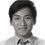0 answersPost by John Zhu on August 12, 2013Yes folks, Ln(0) is never defined! That is a drawing error that SHOULD include a vertical asymptote as Sumant has suggested. Thanks for the feedback and notes guys. Cheers! =)0 answersPost by Him Tam on July 30, 2013Yeah, the graph shouldn't intersect the y-axis0 answersPost by Sumant Nigam on March 8, 2013I thought the video was great, but for the last problem: f(x)=ln(x)-1 the graph should not intersect the y-axis, right? There should be a vertical asymptote there.0 answersPost by Maimouna Louche on June 15, 2012Yey I am first! I loved it all!

### Parametric Curves

• Parametric equation: takes in 2 dependent variables
• Graphing: treat each dependent input variable separately
• Converting to Cartesian equation
• Solve for t in terms of x
• Substitute solved tterm into yterm

### Parametric Curves

Graph f(t) = (3t + 6, cos(t)), for − 2 ≤ t ≤ 2
• Make a table
•  t
 − 2
 − 1
 0
 1
 2
 x(t)
 − 2
 1
 4
 7
 10
 y(t)
 − 0.42
 0.54
 1
 0.54
 − 0.42
Plot the points and graph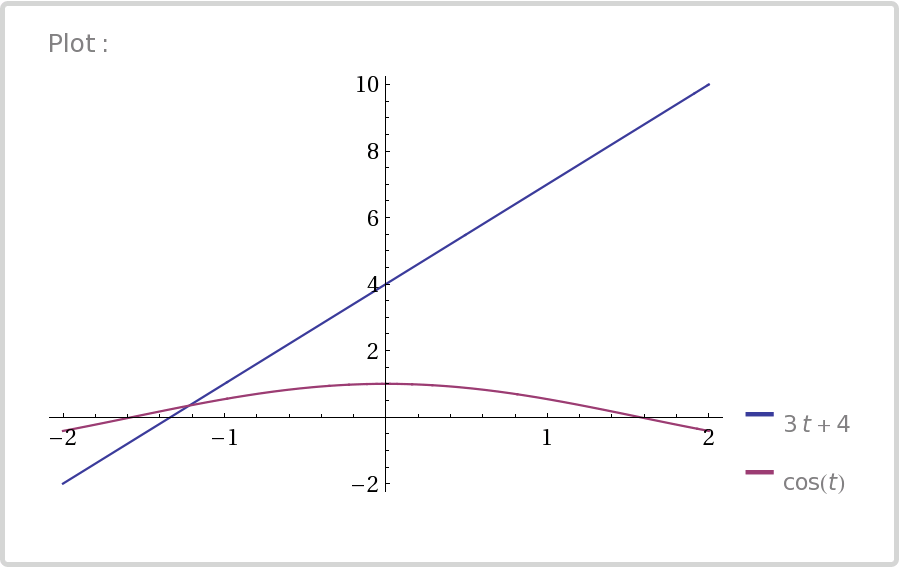Graph f(t) = (sin(x), √x) for 0 ≤ t ≤ 2π
• Make a table
•  t
 0
 2
 π
 [(3π)/2]
 2π
 x(t)
 0
 1
 0
 − 1
 0
 y(t)
 0
 1.25
 1.77
 2.17
 2.51
Plot the points and graph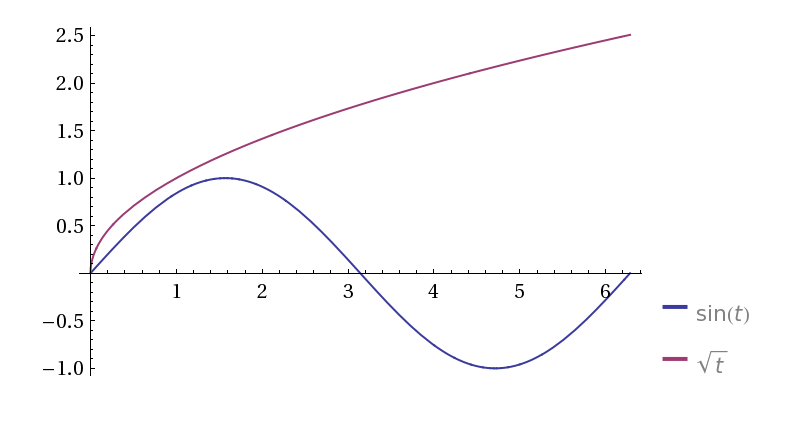A parametric curve is defined by
y(t) = t2
x(t) = t + 2
What is its Cartesian equation?
• Solve for t with x
• x = t + 2
• t = x − 2
Substitute into y(t)
y(t) = t2
y = (x − 2)2
A parametric curve is defined by:
y(t) = t − 9
x(t) = √t
for t ≥ 0
What is its Cartesian equation?
• x = √t
• t = x2
Substitute into y(t)
y(t) = t − 9
y = x2 − 9
Find the Cartesian equation of the following parametric equations:
x(t) = [cos(t)/3]
y(t) = [sin(t)/4]
for t ≥ 0
• Isolate x and y
• x = [cos(t)/3]
• 3x = cos(t)
• y = [sin(t)/4]
• 4y = sin(t)
Apply Pythagorean identity
cos2(t) + sin2(t) = 1
(3x)2 + (4y)2 = 1
9x2 + 16y2 = 1
Find the Cartesian equation of the following parametric equations:
x(t) = √2 sin(t)
y(t) = 2cos(t) + 3
for t ≥ 0
• Isolate x
• x = √2 sin(t)
• [x/(√2 )] = sin(t)
• Isolate y
• y = 2cos(t) + 3
• y − 3 = 2cos(t)
• [(y − 3)/2] = cos(t)
sin2(t) + cos2(t) = 1
([x/(√2 )])2 + ([(y − 3)/2])2 = 1
[(x2)/2] + [((y − 3)2)/4] = 1
Find the Cartesian equation of the following parametric equations:
x(t) = t + 5
y(t) = t3 + 5
• Isolate t with x
• x = t + 5
• x − 5 = t
y = (x − 5)3 + 125
y = x3 − 15x2 + 75x − 125 + 125
y = x3 − 15x2 + 75x
Find the Cartesian equation of the following parametric equations:
x(t) = 1 + t
y(t) = t2 − 9
• Isolate t with x
• x = 1 + t
• − t = 1 − x
• t = x − 1
Substitute into y(t)
y = (x − 1)2 − 9
y = x2 − 2x + 1 − 9
y = x2 − 2x − 8
Find the Cartesian equation of the following parametric equations, and graph it:
x(t) = t2 − 3
y(t) = 1 − t
for t − 3
• Isolate t with x
• x = t2 − 3
• x + 3 = t2
• √{x + 3} = t
• Substitute into y(t)
• y = 1 − √{x + 3}
• Graph the equation using shifts
• y = 1 − √{x + 3}
The graph √x has been shifted up by 1 unit to y = 1, and left by 3 units. It also has been reflected.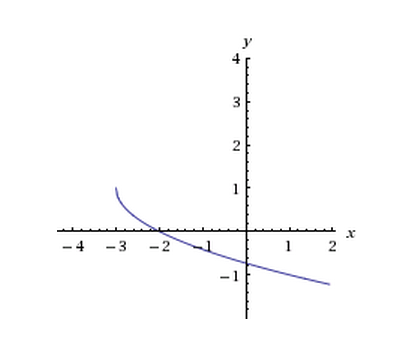Find the Cartesian equation of the following parametric equations, and graph it:
x(t) = arccos([t/4]) − [(π)/2]
y(t) = [t/8] − 3 for − 2π ≤ t ≤ 2π
• Isolate t with x
• x = arccos([t/4]) − [(π)/2]
• x + [(π)/2] = arccos([t/4])
• cos(x + [(π)/2]) = [t/4]
• 4cos(x + [(π)/2]) = t
• Observe half - angle trig identity
• cos(u + [(π)/2]) = − sinu
• 4cos(x + [(π)/2]) = t
• − 4sinx = t
• Substitute into y(t)
• y = [( − 4sin(x))/8] − 3
• y = − [sinx/2] − 3
• Graph using shifts, reflections, and stretching
• y = − [sinx/2] − 3
• The graph y = sinx has been shifted 3 units down to y = − 3, and reflected. It also has been compressed by a factor of 2.
• Remember to graph within the bounds − 2π ≤ t ≤ 2π.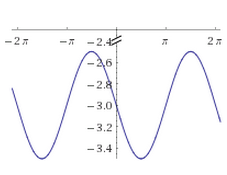*These practice questions are only helpful when you work on them offline on a piece of paper and then use the solution steps function to check your answer.

### Parametric Curves

Lecture Slides are screen-captured images of important points in the lecture. Students can download and print out these lecture slide images to do practice problems as well as take notes while watching the lecture.

• Intro 0:00
• Parametric Equations 0:23
• Familiar Functions
• Parametric Equation/ Function
• Example 1: Graph Parametric Equation 1:48
• Example 2 4:30
• Example 3 6:01
• Example 4 7:12
• Example 5 8:10

OR

### Start Learning Now

Our free lessons will get you started (Adobe Flash® required).